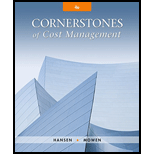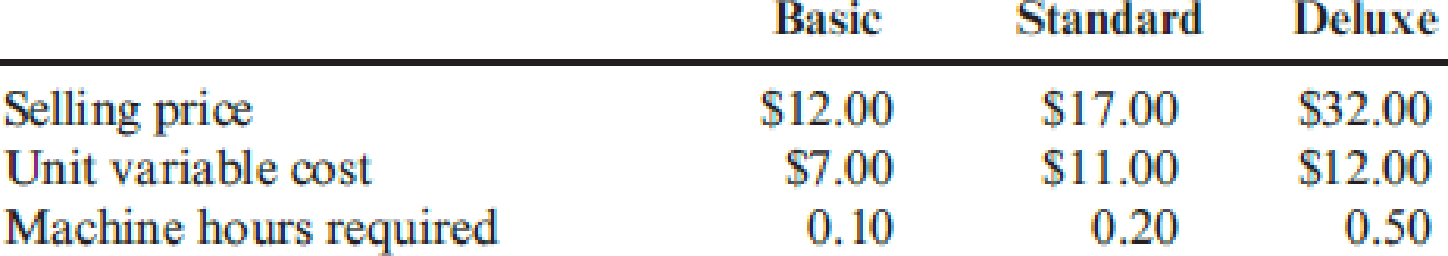# Behar Company makes three types of stainless steel frying pans. Each of the three types of pans requires the use of a special machine that has total operating capacity of 182,000 hours per year. Information on each of the three products is as follows: The marketing manager has determined that the company can sell all that it can produce of each of the three products. Required: 1. How many of each product should be sold to maximize the total contribution margin? What is the total contribution margin for this product mix? 2. Suppose that Behar can sell no more than 300,000 units of each type at the prices indicated. What product mix would you recommend, and what would be the total contribution margin?### Cornerstones of Cost Management (C...

4th Edition
Don R. Hansen + 1 other
Publisher: Cengage Learning
ISBN: 9781305970663

#### Solutions

Chapter
Section### Cornerstones of Cost Management (C...

4th Edition
Don R. Hansen + 1 other
Publisher: Cengage Learning
ISBN: 9781305970663
Chapter 20, Problem 16E
Textbook Problem
89 views

## Behar Company makes three types of stainless steel frying pans. Each of the three types of pans requires the use of a special machine that has total operating capacity of 182,000 hours per year. Information on each of the three products is as follows:The marketing manager has determined that the company can sell all that it can produce of each of the three products.Required: 1. How many of each product should be sold to maximize the total contribution margin? What is the total contribution margin for this product mix? 2. Suppose that Behar can sell no more than 300,000 units of each type at the prices indicated. What product mix would you recommend, and what would be the total contribution margin?

1.

To determine

Ascertain the number of each product that should be sold to maximize the total contribution margin and compute the total contribution margin for the given product mix.

### Explanation of Solution

Contribution margin: Contribution margin is a measurement of performance where only revenue and variable costs are taken into consideration. Hence, this measurement is useful in the evaluation of the probable outcomes of decisions including pricing decisions and other marketing strategies that affect primarily revenue and variable costs.

Ascertain the number of each product that should be sold to maximize the total contribution margin:

 Particulars Basic Standard Deluxe Price $12.00$17.00 $32.00 Less: variable cost 7.00 11.00 12.00 Contribution margin (a)$5.00 $6.00$20.00 Machine hours (b) 0.10 0.20 0.50 Contribution margin per machine hour (a×b) \$50

2.

To determine

State the product mix that is recommendable, and compute the total contribution margin for the product mix.

### Still sussing out bartleby?

Check out a sample textbook solution.

See a sample solution

#### The Solution to Your Study Problems

Bartleby provides explanations to thousands of textbook problems written by our experts, many with advanced degrees!

Get Started

Find more solutions based on key concepts
What are the major components of a marketing strategy?

Foundations of Business (MindTap Course List)

EXPECTED AND REQUIRED RATES OF RETURN Assume that the risk-free rate is 5% and the market risk premium is 6%. W...

Fundamentals of Financial Management, Concise Edition (with Thomson ONE - Business School Edition, 1 term (6 months) Printed Access Card) (MindTap Course List)

Explain the relationship between NPV and a firms value.

Managerial Accounting: The Cornerstone of Business Decision-Making